|

# 35㎡蜗居,爆改后惊艳变身一室一厅有花有草的小复式!

其实房君是个俗气的人，见山喜山，见海喜海，见胡歌喜胡歌，见彭于晏喜彭于晏，却在这二十几个年头中，一直深爱着loft，尤其是原木风的loft。这个故事的开头，就要从上海永康路38弄46号的一个老房子说起。

这里在旧时是上海的法租界，弄堂中的日子过的总是很缓慢，老房子的灰尘堆积起来，都能绕过百年的时光。先来一张史前巨坑的房屋全景图吧。

斑驳的墙面，脱落的墙皮，快要腐蚀的木家具，每一件都在诉说着上个世纪，在这屋子里发生的，或感动或狗血的故事。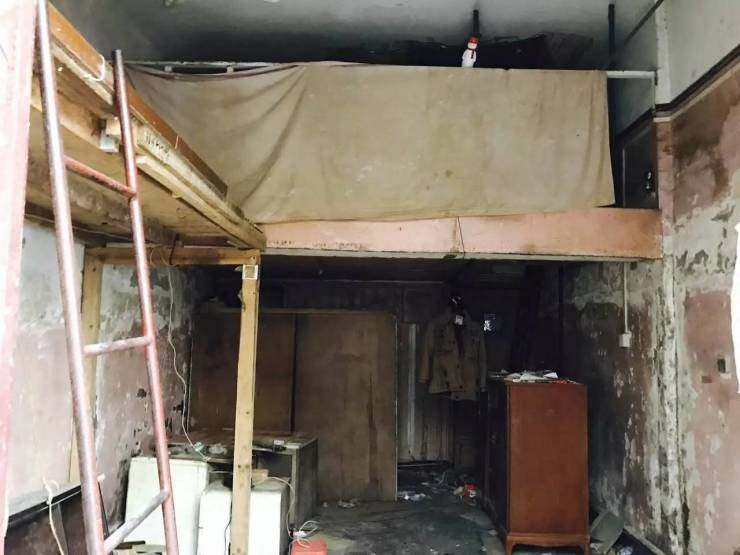沭阳从房东手里接过这个他都记不清是哪一年的房子时，心里是有些雀跃的，虽然房子整体特别破旧，但也正是这种环境，让她能施展拳脚好好爆改这个房子。

让那些流淌在上个世纪的故事，都变成自己的故事。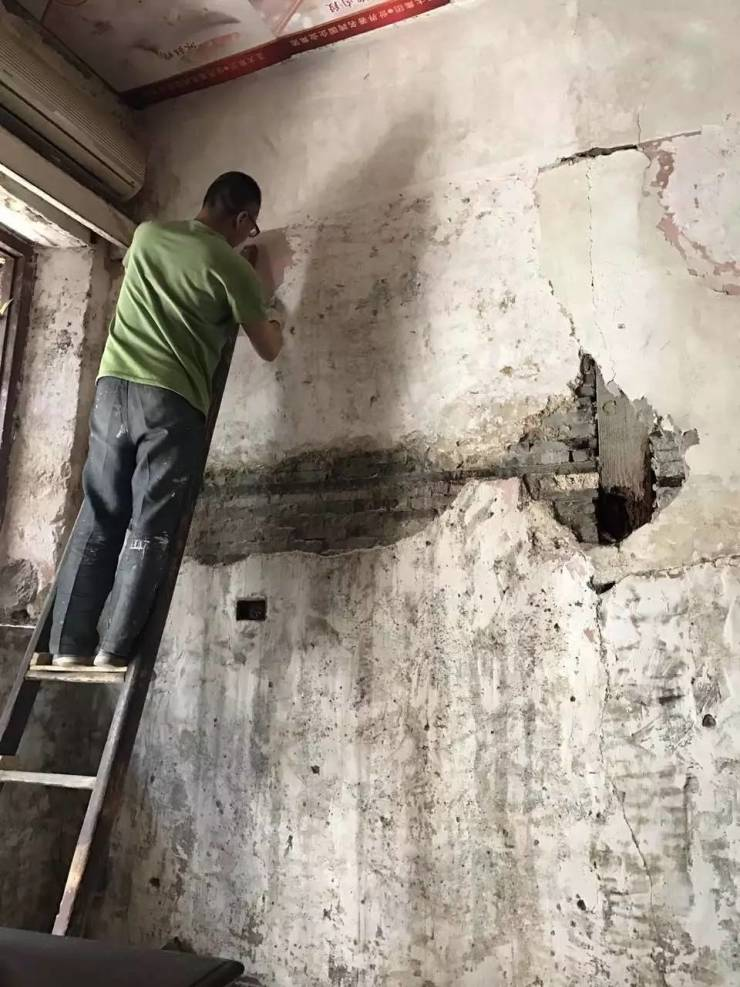高挑的空间可以做点有意思的东西，比如，loft。

要说小户型神器，就是loft和榻榻米了，但是loft能把每个空间独立出来，这点比榻榻米要实惠些。

所以直接在墙角的部分加个小复式，一层变两层，二楼是客厅一楼是卧室，这个只有35㎡左右的小房子，也变成一室一厅了。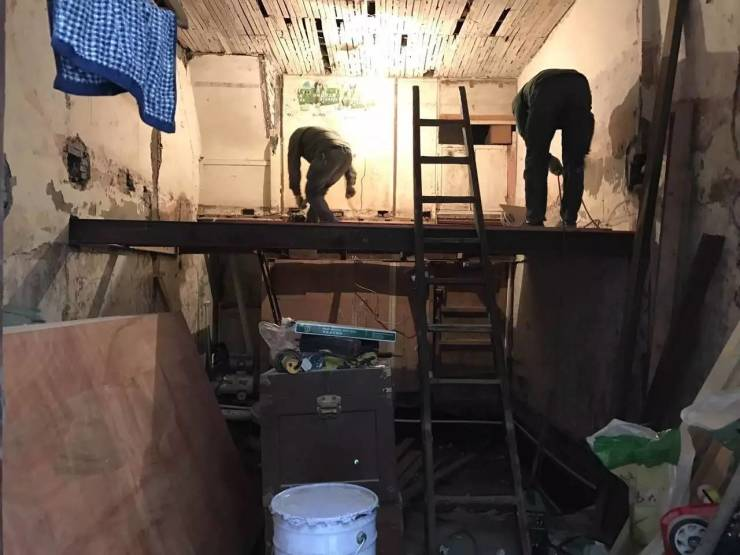两个月后，这个屋子就再也不复当年凄惨的模样了。

玄关

▽

沭阳本想淘一个扎染布的，可是在她看到这个猫头鹰的时候，就一见倾心，好像在今世，遇见了前世回眸好几百遍的人般心动。

这个用木头干草枯叶手工做成的猫头鹰，好像在问她：嘿，美女，去霍格沃茨吗。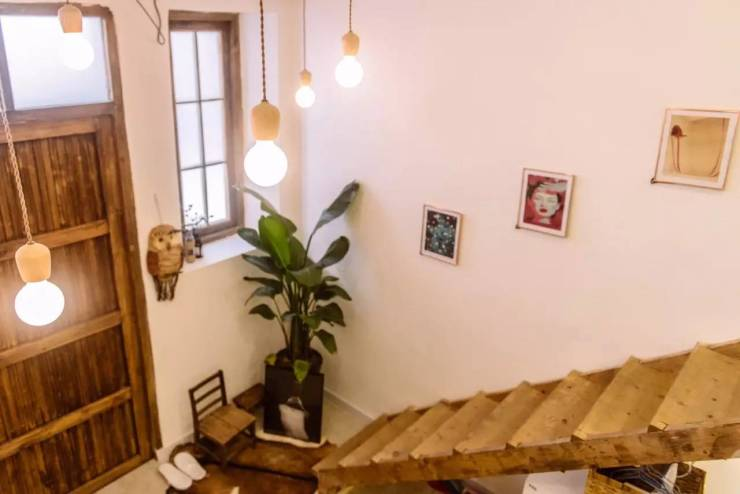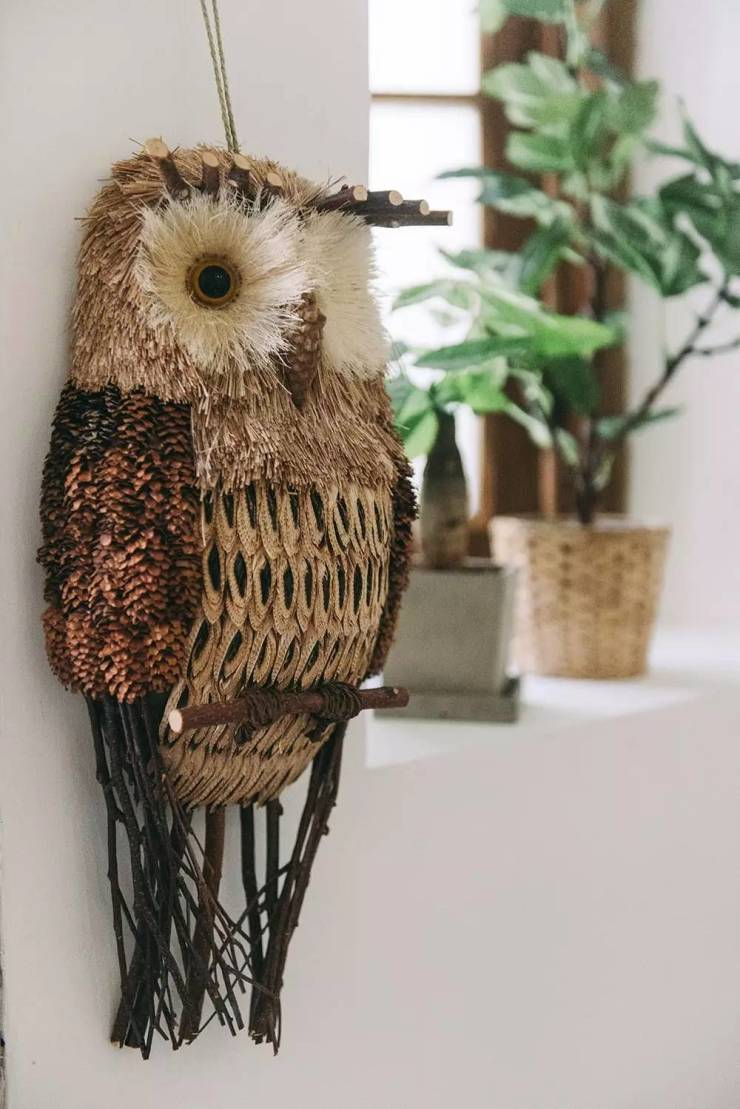厨房

▽

厨房就在进门处，和玄关相对，因为东西不多所以没有选择收纳柜，而是在墙上安装了几块搁板，厨房器具和装饰品都摆在上面。

因为器具的样式很漂亮，和装饰品摆在一起，也分不清究竟谁是烟火，谁是艺术。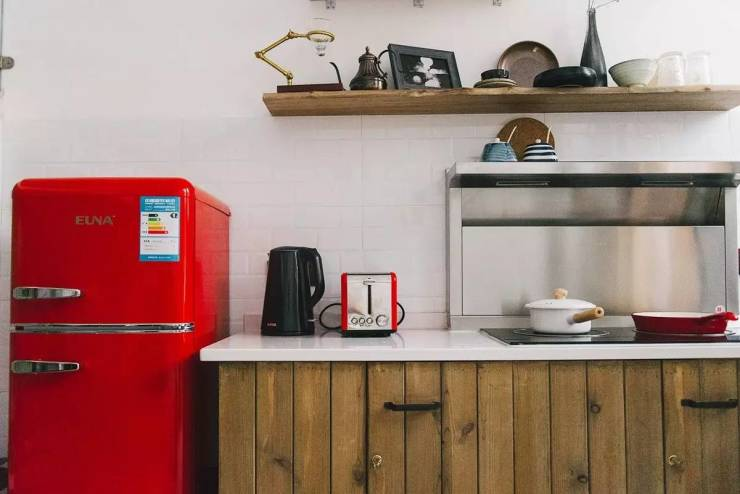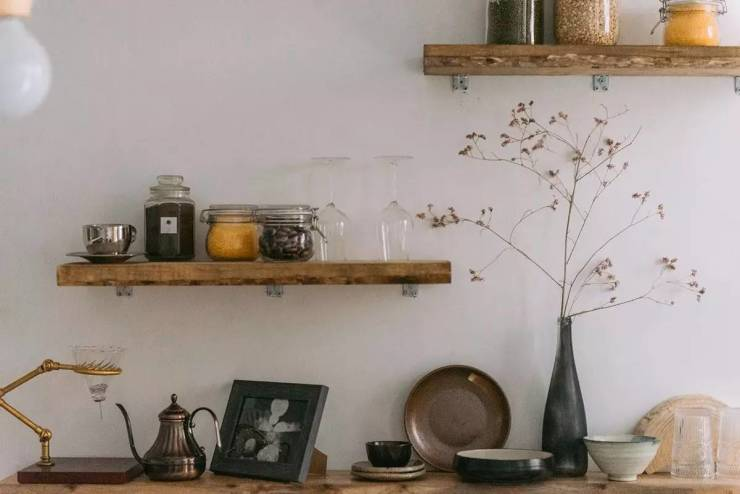炉灶也没有选择传统的炉灶，而是电磁灶。

电磁灶已经走进了越来越多的年轻人家中，它的缺点是火力有限，传统灶台明火做产生的温度在500度以上，中餐里讲究的大火力高温，普通的电磁灶是望尘莫及的。

但是电磁灶的优点，是更多现代人所需要的，它热效率快，节能节气，而且容易维护，不容易烫伤手，同时还不存在煤气泄漏的安全问题，最重要的是，颜值高！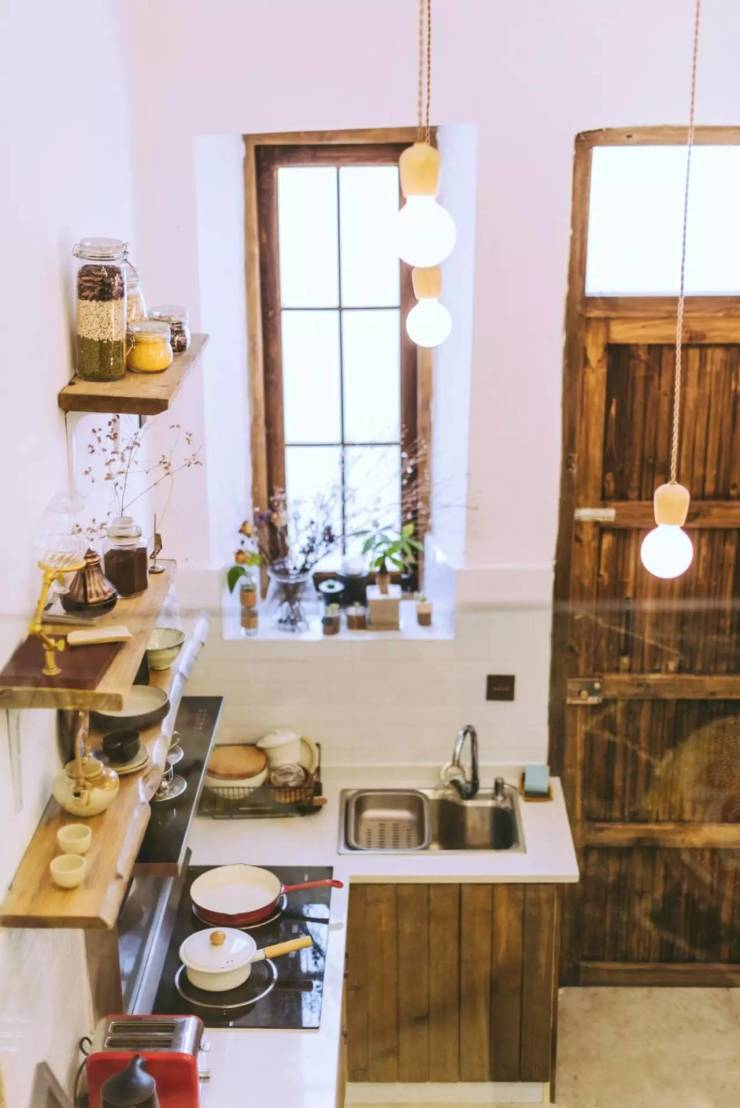卧室

▽

沭阳改变了大多数Loft一楼客厅二楼卧室的格局，把卧室放在了一楼。

因为老房子的隔音不好是无法解决的，卧室在一楼可缓解隔音问题，同时晚上去卫生间也更方便。双人窗边有一个木质的小衣架，可以把衣服挂在上边，也可以挂一盏灯。

墙面也没有什么过多的修饰，挂一个流苏，和同色系的钟表，不愿意上楼吃饭的时候可以直接在右边的木桌子上吃，但平时它就是一个工作台。洗手池直接放在床的对面，毛巾什么的挂在墙上。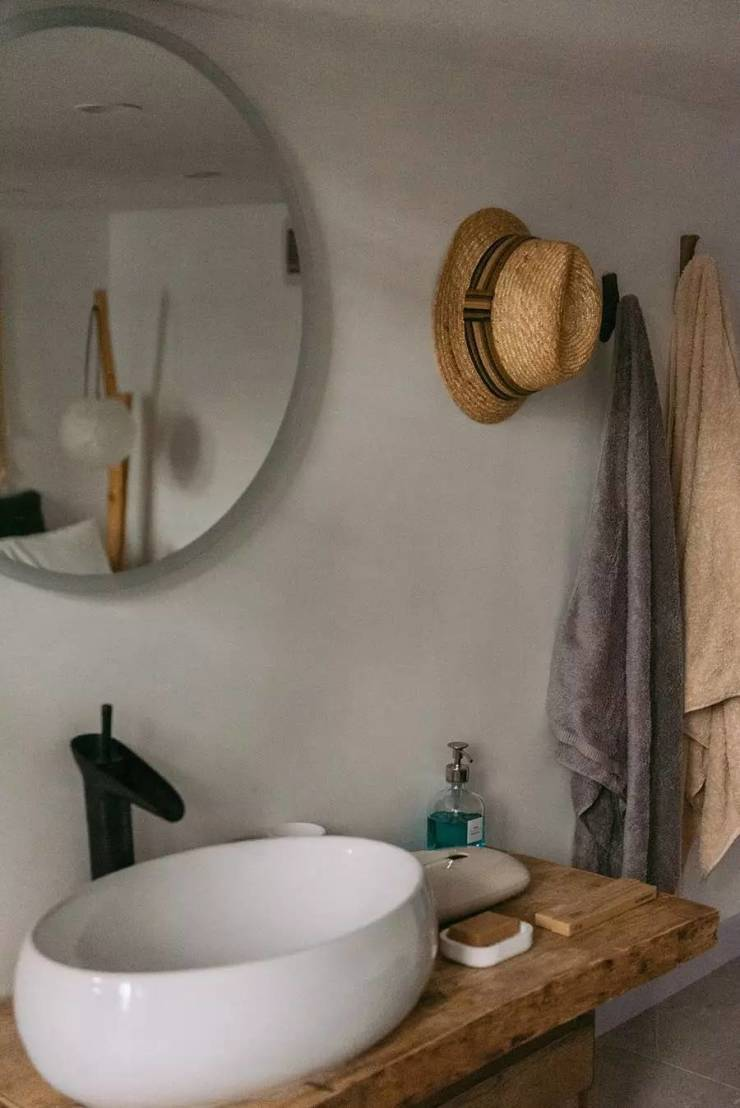当然洗手间还是独立出来的，但是因为面积太小， 不如直接把洗手池搬出来。休闲区域

▽

二楼的休闲区域，用的是色彩浓烈的民族风格，就类似于人的两面性，喜欢热闹繁华，又喜欢安静自我。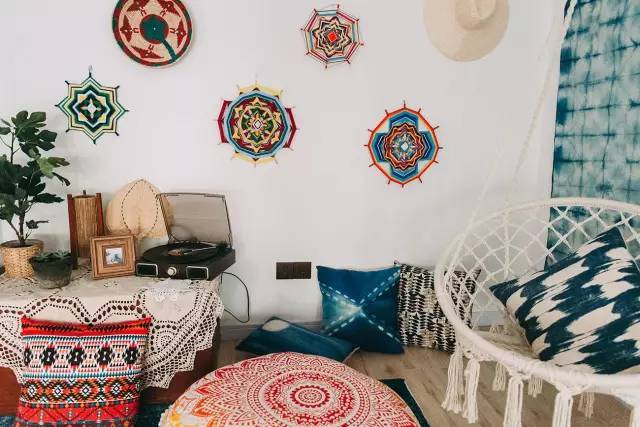在浓烈的色彩下，依然有质朴的木，和充满老上海风情的唱片机。

阳光热烈的午后，三五好友在房间里聊天调酒看电影，听着喜欢的唱片，一边轻声跟着哼唱，一边眯着眼睛虚度时光。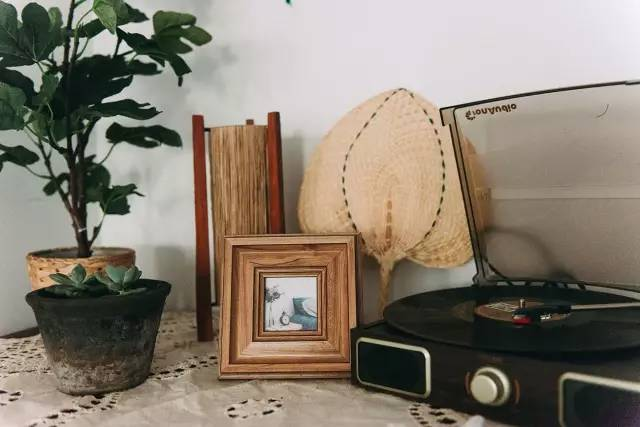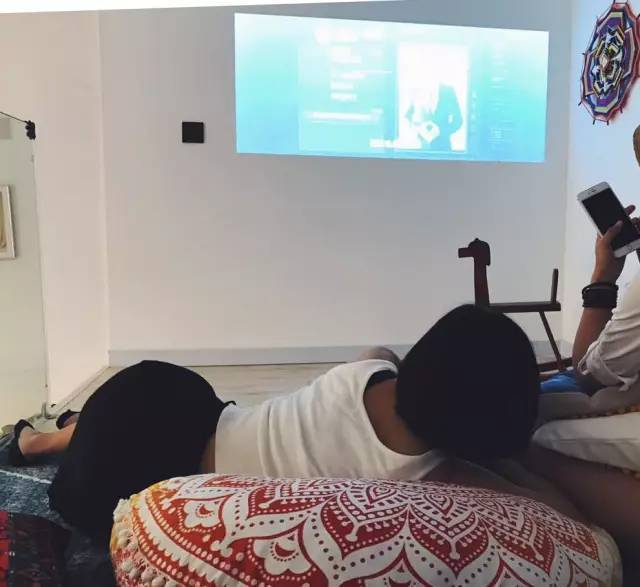墙上的曼达拉是沭阳亲手编织的，编织每一个都要6小时以上。

如此心灵手巧之人，咳咳，房君话至此处大家自行领悟。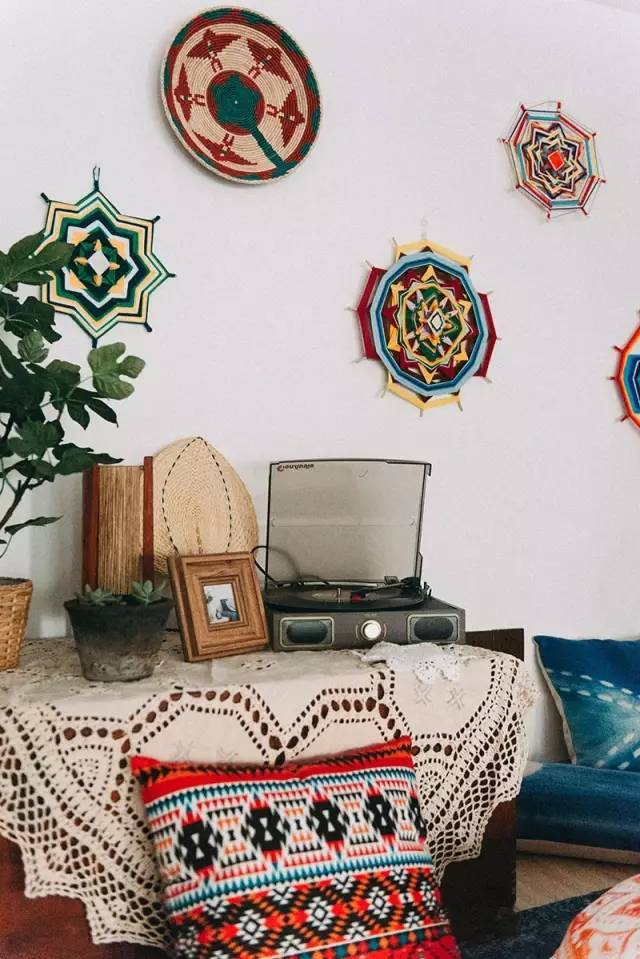沭阳的好友是调酒师，也是咖啡爱好者，她们说，「欢迎大家来我们的房子，我们可以愉快的聊天，还可以泡咖啡或调酒给你们喝」。至于为什么把屋子取名为「瞳孔」，是因为，「孔中乾坤大，孔中岁月长」。

眼睛是万物之源，瞳孔虽小却包含着整个世界。

欢迎你来到沭阳的世界，也谢谢你的到来，让她看到不一样的世界。

其实「瞳孔」是个民宿，但是它和很多比较奢华、冷淡的民宿都不一样，除了在极具情怀的弄堂里，这个民宿被沭阳打造的，像个真正的温暖的家。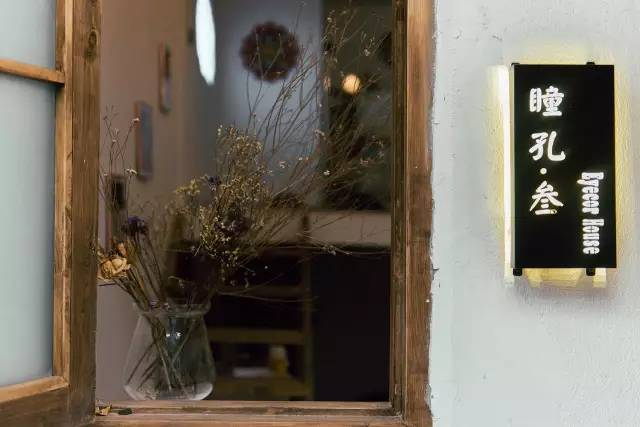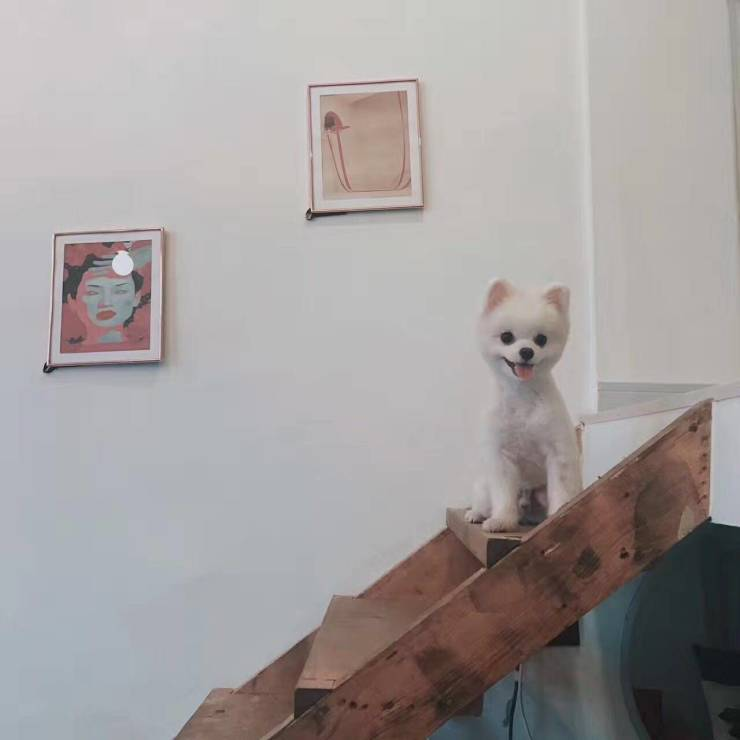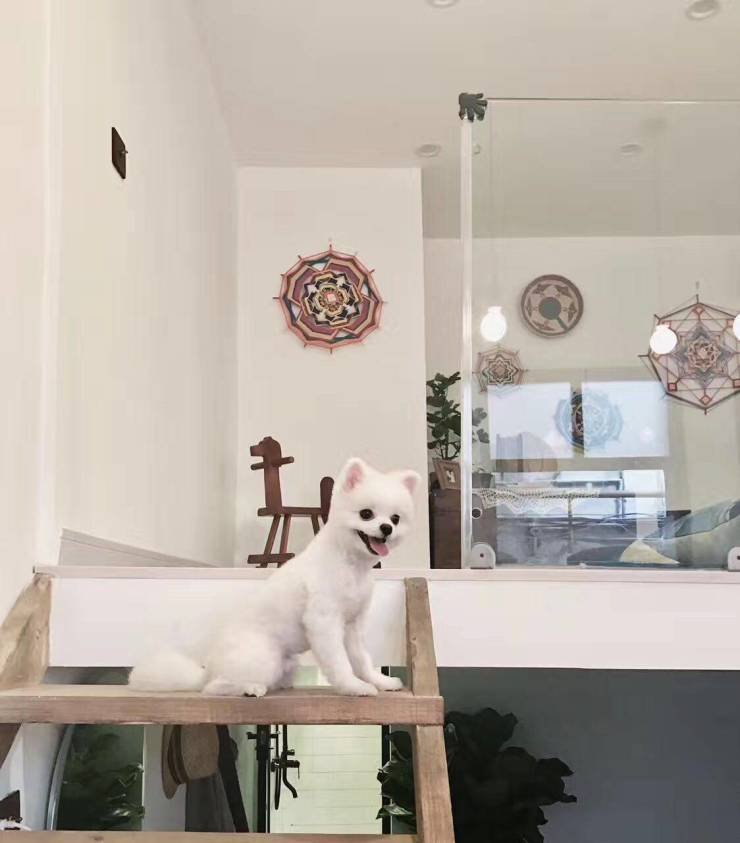▲运气好的话，还能遇见隔壁纹身店的狗狗，肿么办，看到这只狗，房君感到了深深的绝望，云吸狗已经快无法满足我了QAQ

「瞳孔」所有的木头，都是淘来的旧木料，以旧木为主，搭配粗陶和干花，给人以安静淡然的气氛。

就好像，年少时期的我们，大约都曾渴望过命运的波澜，但到现在发现，人生最曼妙的风景，其实是是内心的淡定与从容。

`声明：本文由入驻焦点开放平台的作者撰写，除焦点官方账号外，观点仅代表作者本人，不代表焦点立场错误信息举报电话： 400-099-0099，邮箱：jubao@vip.sohu.com，或点此进行意见反馈，或点此进行举报投诉。`A B C D E F G H J K L M N P Q R S T W X Y Z
A - B - C - D - E
• A
• 鞍山
• 安庆
• 安阳
• 安顺
• 安康
• 澳门
• B
• 北京
• 保定
• 包头
• 巴彦淖尔
• 本溪
• 蚌埠
• 亳州
• 滨州
• 北海
• 百色
• 巴中
• 毕节
• 保山
• 宝鸡
• 白银
• 巴州
• C
• 承德
• 沧州
• 长治
• 赤峰
• 朝阳
• 长春
• 常州
• 滁州
• 池州
• 长沙
• 常德
• 郴州
• 潮州
• 崇左
• 重庆
• 成都
• 楚雄
• 昌都
• 慈溪
• 常熟
• D
• 大同
• 大连
• 丹东
• 大庆
• 东营
• 德州
• 东莞
• 德阳
• 达州
• 大理
• 德宏
• 定西
• 儋州
• 东平
• E
• 鄂尔多斯
• 鄂州
• 恩施
F - G - H - I - J
• F
• 抚顺
• 阜新
• 阜阳
• 福州
• 抚州
• 佛山
• 防城港
• G
• 赣州
• 广州
• 桂林
• 贵港
• 广元
• 广安
• 贵阳
• 固原
• H
• 邯郸
• 衡水
• 呼和浩特
• 呼伦贝尔
• 葫芦岛
• 哈尔滨
• 黑河
• 淮安
• 杭州
• 湖州
• 合肥
• 淮南
• 淮北
• 黄山
• 菏泽
• 鹤壁
• 黄石
• 黄冈
• 衡阳
• 怀化
• 惠州
• 河源
• 贺州
• 河池
• 海口
• 红河
• 汉中
• 海东
• I
• J
• 晋中
• 锦州
• 吉林
• 鸡西
• 佳木斯
• 嘉兴
• 金华
• 景德镇
• 九江
• 吉安
• 济南
• 济宁
• 焦作
• 荆门
• 荆州
• 江门
• 揭阳
• 金昌
• 酒泉
• 嘉峪关
K - L - M - N - P
• K
• 开封
• 昆明
• 昆山
• L
• 廊坊
• 临汾
• 辽阳
• 连云港
• 丽水
• 六安
• 龙岩
• 莱芜
• 临沂
• 聊城
• 洛阳
• 漯河
• 娄底
• 柳州
• 来宾
• 泸州
• 乐山
• 六盘水
• 丽江
• 临沧
• 拉萨
• 林芝
• 兰州
• 陇南
• M
• 牡丹江
• 马鞍山
• 茂名
• 梅州
• 绵阳
• 眉山
• N
• 南京
• 南通
• 宁波
• 南平
• 宁德
• 南昌
• 南阳
• 南宁
• 内江
• 南充
• P
• 盘锦
• 莆田
• 平顶山
• 濮阳
• 攀枝花
• 普洱
• 平凉
Q - R - S - T - W
• Q
• 秦皇岛
• 齐齐哈尔
• 衢州
• 泉州
• 青岛
• 清远
• 钦州
• 黔南
• 曲靖
• 庆阳
• R
• 日照
• 日喀则
• S
• 石家庄
• 沈阳
• 双鸭山
• 绥化
• 上海
• 苏州
• 宿迁
• 绍兴
• 宿州
• 三明
• 上饶
• 三门峡
• 商丘
• 十堰
• 随州
• 邵阳
• 韶关
• 深圳
• 汕头
• 汕尾
• 三亚
• 三沙
• 遂宁
• 山南
• 商洛
• 石嘴山
• T
• 天津
• 唐山
• 太原
• 通辽
• 铁岭
• 泰州
• 台州
• 铜陵
• 泰安
• 铜仁
• 铜川
• 天水
• 天门
• W
• 乌海
• 乌兰察布
• 无锡
• 温州
• 芜湖
• 潍坊
• 威海
• 武汉
• 梧州
• 渭南
• 武威
• 吴忠
• 乌鲁木齐
X - Y - Z
• X
• 邢台
• 徐州
• 宣城
• 厦门
• 新乡
• 许昌
• 信阳
• 襄阳
• 孝感
• 咸宁
• 湘潭
• 湘西
• 西双版纳
• 西安
• 咸阳
• 西宁
• 仙桃
• 西昌
• Y
• 运城
• 营口
• 盐城
• 扬州
• 鹰潭
• 宜春
• 烟台
• 宜昌
• 岳阳
• 益阳
• 永州
• 阳江
• 云浮
• 玉林
• 宜宾
• 雅安
• 玉溪
• 延安
• 榆林
• 银川
• Z
• 张家口
• 镇江
• 舟山
• 漳州
• 淄博
• 枣庄
• 郑州
• 周口
• 驻马店
• 株洲
• 张家界
• 珠海
• 湛江
• 肇庆
• 中山
• 自贡
• 资阳
• 遵义
• 昭通
• 张掖
• 中卫

1室1厅1厨1卫1阳台

1
2
3
4
5

0
1
2

1

1

0
1
2
3报名成功，资料已提交审核A B C D E F G H J K L M N P Q R S T W X Y Z
A - B - C - D - E
• A
• 鞍山
• 安庆
• 安阳
• 安顺
• 安康
• 澳门
• B
• 北京
• 保定
• 包头
• 巴彦淖尔
• 本溪
• 蚌埠
• 亳州
• 滨州
• 北海
• 百色
• 巴中
• 毕节
• 保山
• 宝鸡
• 白银
• 巴州
• C
• 承德
• 沧州
• 长治
• 赤峰
• 朝阳
• 长春
• 常州
• 滁州
• 池州
• 长沙
• 常德
• 郴州
• 潮州
• 崇左
• 重庆
• 成都
• 楚雄
• 昌都
• 慈溪
• 常熟
• D
• 大同
• 大连
• 丹东
• 大庆
• 东营
• 德州
• 东莞
• 德阳
• 达州
• 大理
• 德宏
• 定西
• 儋州
• 东平
• E
• 鄂尔多斯
• 鄂州
• 恩施
F - G - H - I - J
• F
• 抚顺
• 阜新
• 阜阳
• 福州
• 抚州
• 佛山
• 防城港
• G
• 赣州
• 广州
• 桂林
• 贵港
• 广元
• 广安
• 贵阳
• 固原
• H
• 邯郸
• 衡水
• 呼和浩特
• 呼伦贝尔
• 葫芦岛
• 哈尔滨
• 黑河
• 淮安
• 杭州
• 湖州
• 合肥
• 淮南
• 淮北
• 黄山
• 菏泽
• 鹤壁
• 黄石
• 黄冈
• 衡阳
• 怀化
• 惠州
• 河源
• 贺州
• 河池
• 海口
• 红河
• 汉中
• 海东
• I
• J
• 晋中
• 锦州
• 吉林
• 鸡西
• 佳木斯
• 嘉兴
• 金华
• 景德镇
• 九江
• 吉安
• 济南
• 济宁
• 焦作
• 荆门
• 荆州
• 江门
• 揭阳
• 金昌
• 酒泉
• 嘉峪关
K - L - M - N - P
• K
• 开封
• 昆明
• 昆山
• L
• 廊坊
• 临汾
• 辽阳
• 连云港
• 丽水
• 六安
• 龙岩
• 莱芜
• 临沂
• 聊城
• 洛阳
• 漯河
• 娄底
• 柳州
• 来宾
• 泸州
• 乐山
• 六盘水
• 丽江
• 临沧
• 拉萨
• 林芝
• 兰州
• 陇南
• M
• 牡丹江
• 马鞍山
• 茂名
• 梅州
• 绵阳
• 眉山
• N
• 南京
• 南通
• 宁波
• 南平
• 宁德
• 南昌
• 南阳
• 南宁
• 内江
• 南充
• P
• 盘锦
• 莆田
• 平顶山
• 濮阳
• 攀枝花
• 普洱
• 平凉
Q - R - S - T - W
• Q
• 秦皇岛
• 齐齐哈尔
• 衢州
• 泉州
• 青岛
• 清远
• 钦州
• 黔南
• 曲靖
• 庆阳
• R
• 日照
• 日喀则
• S
• 石家庄
• 沈阳
• 双鸭山
• 绥化
• 上海
• 苏州
• 宿迁
• 绍兴
• 宿州
• 三明
• 上饶
• 三门峡
• 商丘
• 十堰
• 随州
• 邵阳
• 韶关
• 深圳
• 汕头
• 汕尾
• 三亚
• 三沙
• 遂宁
• 山南
• 商洛
• 石嘴山
• T
• 天津
• 唐山
• 太原
• 通辽
• 铁岭
• 泰州
• 台州
• 铜陵
• 泰安
• 铜仁
• 铜川
• 天水
• 天门
• W
• 乌海
• 乌兰察布
• 无锡
• 温州
• 芜湖
• 潍坊
• 威海
• 武汉
• 梧州
• 渭南
• 武威
• 吴忠
• 乌鲁木齐
X - Y - Z
• X
• 邢台
• 徐州
• 宣城
• 厦门
• 新乡
• 许昌
• 信阳
• 襄阳
• 孝感
• 咸宁
• 湘潭
• 湘西
• 西双版纳
• 西安
• 咸阳
• 西宁
• 仙桃
• 西昌
• Y
• 运城
• 营口
• 盐城
• 扬州
• 鹰潭
• 宜春
• 烟台
• 宜昌
• 岳阳
• 益阳
• 永州
• 阳江
• 云浮
• 玉林
• 宜宾
• 雅安
• 玉溪
• 延安
• 榆林
• 银川
• Z
• 张家口
• 镇江
• 舟山
• 漳州
• 淄博
• 枣庄
• 郑州
• 周口
• 驻马店
• 株洲
• 张家界
• 珠海
• 湛江
• 肇庆
• 中山
• 自贡
• 资阳
• 遵义
• 昭通
• 张掖
• 中卫• 手机• 分享
• 设计
免费设计
• 计算器
装修计算器
• 入驻
合作入驻
• 联系
联系我们
• 置顶
返回顶部